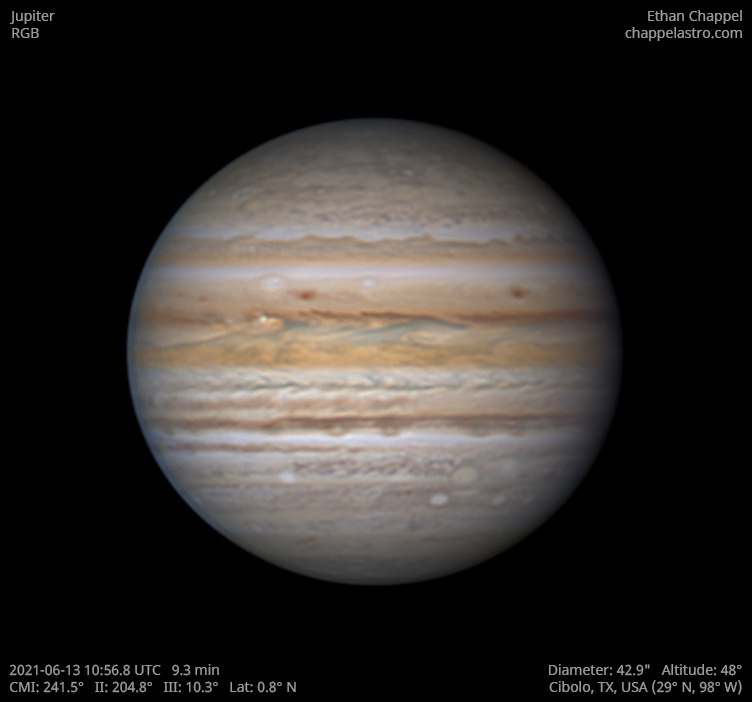# Jupiter 2021-06-13 10:56 UTC

CM1: 241.50°

CM2: 204.80°

CM3: 10.30°

CLat: +0.80°

Description

Excellent seeing conditions. This is a second attempt with my EdgeHD 11 and new Sky-Watcher EQ6-R Pro after a near-failure the previous morning because of clouds. My primary use for this mount will be for deep sky imaging, but I can also use it for planetary imaging with the EdgeHD 11 in times I would've used the EdgeHD 8 and Advanced VX.

Notes:

• Both the North & South Equatorial Belts appear faded except for their southern edges.
• The Equatorial Zone, except for the southern edge, is still orange.
• Oval BA has a slight orange color.

Equipment

ZWO ASI290MM

Celestron EdgeHD 11

Sky-Watcher EQ6-R Pro

Logs
```FireCapture v2.7 04 BETA Settings
------------------------------------
Observer=Ethan Chappel
Location=Cibolo
Scope=Celestron C8 EdgeHD
Camera=ZWO ASI290MM
Filter=B
Profile=Jupiter
Diameter=42.83"
Magnitude=-2.52
CMI=243.0° CMII=206.3° CMIII=11.8°  (during mid of capture)
FocalLength=4450mm (F/21)
Resolution=0.13"
Filename=j2021-06-13_11-00-13_b_ec.ser
Date=2021_06_13
Start=10_58_58.906
Mid=11_00_13.904
End=11_01_28.903
Start(UT)=10_58_58.906
Mid(UT)=11_00_13.904
End(UT)=11_01_28.903
Duration=149.997s
Date_format=yyyy_MM_dd
Time_format=HH_mm_ss
LT=UT
Frames captured=16832
File type=SER
Binning=1x1
ROI=432x414
ROI(Offset)=1080x248
FPS (avg.)=112
Shutter=8.000ms
Gain=350 (58%)
AutoExposure=off
AutoGain=off
Brightness=1
HighSpeed=on
USBTraffic=50
Histogramm(min)=0
Histogramm(max)=201
Histogramm=78%
Noise(avg.deviation)=1.78
Limit=150 Seconds
Sensor temperature=96.1°F
FireCapture v2.7 04 BETA Settings
------------------------------------
Observer=Ethan Chappel
Location=Cibolo
Scope=Celestron C8 EdgeHD
Camera=ZWO ASI290MM
Filter=eG
Profile=Jupiter
Diameter=42.83"
Magnitude=-2.52
CMI=240.9° CMII=204.2° CMIII=9.7°  (during mid of capture)
FocalLength=4400mm (F/21)
Resolution=0.14"
Filename=j2021-06-13_10-56-48_eg_ec.ser
Date=2021_06_13
Start=10_55_33.922
Mid=10_56_48.923
End=10_58_03.924
Start(UT)=10_55_33.922
Mid(UT)=10_56_48.923
End(UT)=10_58_03.924
Duration=150.002s
Date_format=yyyy_MM_dd
Time_format=HH_mm_ss
LT=UT
Frames captured=18742
File type=SER
Binning=1x1
ROI=432x414
ROI(Offset)=1080x248
FPS (avg.)=124
Shutter=8.000ms
Gain=300 (50%)
AutoExposure=off
AutoGain=off
Brightness=1
HighSpeed=on
USBTraffic=100
Histogramm(min)=0
Histogramm(max)=197
Histogramm=77%
Noise(avg.deviation)=1.78
Limit=150 Seconds
Sensor temperature=96.1°F
FireCapture v2.7 04 BETA Settings
------------------------------------
Observer=Ethan Chappel
Location=Cibolo
Scope=Celestron C8 EdgeHD
Camera=ZWO ASI290MM
Filter=R
Profile=Jupiter
Diameter=42.83"
Magnitude=-2.52
CMI=238.9° CMII=202.2° CMIII=7.7°  (during mid of capture)
FocalLength=4450mm (F/21)
Resolution=0.14"
Filename=j2021-06-13_10-53-26_r_ec.ser
Date=2021_06_13
Start=10_52_11.050
Mid=10_53_26.048
End=10_54_41.046
Start(UT)=10_52_11.050
Mid(UT)=10_53_26.048
End(UT)=10_54_41.046
Duration=149.996s
Date_format=yyyy_MM_dd
Time_format=HH_mm_ss
LT=UT
Frames captured=16832
File type=SER
Binning=1x1
ROI=432x414
ROI(Offset)=1080x248
FPS (avg.)=112
Shutter=8.000ms
Gain=300 (50%)
AutoExposure=off
AutoGain=off
Brightness=1
HighSpeed=on
USBTraffic=50
Histogramm(min)=0
Histogramm(max)=192
Histogramm=75%
Noise(avg.deviation)=1.34
Limit=150 Seconds
Sensor temperature=96.8°F
```